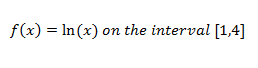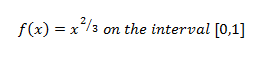Clutch Prep is now a part of Pearson
Ch.8: Applications of Derivatives (Part 1)WorksheetSee all chapters

# Theorems (MVT)

See all sections
Sections
Implicit Differentiation
Related Rates
Theorems (MVT)

Concept #1

Concept #2: Intro

Practice: Verify that the hypothesis of the Mean-Value Theorem is satisfied on the given interval. Find all the values of c in the interval that satisfies the conclusion of the theorem.Practice: Verify that the hypothesis of the Mean-Value Theorem is satisfied on the given interval. Find all the values of c in the interval that satisfies the conclusion of the theorem.Concept #3: Rolle's Theorem